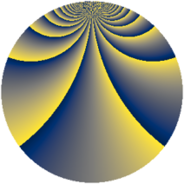# Properties

 Label 1336.2.iLevel $1336$ Weight $2$ Character orbit 1336.i Rep. character $\chi_{1336}(9,\cdot)$ Character field $\Q(\zeta_{83})$ Dimension $3444$ Sturm bound $336$

# Related objects

## Defining parameters

 Level: $$N$$ $$=$$ $$1336 = 2^{3} \cdot 167$$ Weight: $$k$$ $$=$$ $$2$$ Character orbit: $$[\chi]$$ $$=$$ 1336.i (of order $$83$$ and degree $$82$$) Character conductor: $$\operatorname{cond}(\chi)$$ $$=$$ $$167$$ Character field: $$\Q(\zeta_{83})$$ Sturm bound: $$336$$

## Dimensions

The following table gives the dimensions of various subspaces of $$M_{2}(1336, [\chi])$$.

Total New Old
Modular forms 14104 3444 10660
Cusp forms 13448 3444 10004
Eisenstein series 656 0 656

## Trace form

 $$3444q + 2q^{5} - 38q^{9} + O(q^{10})$$ $$3444q + 2q^{5} - 38q^{9} + 4q^{11} + 2q^{13} + 8q^{17} + 4q^{19} - 42q^{25} + 12q^{27} - 8q^{29} + 8q^{31} + 8q^{33} + 12q^{35} + 2q^{37} - 16q^{39} + 4q^{41} + 2q^{43} + 10q^{45} - 4q^{47} - 38q^{49} - 24q^{51} + 6q^{53} - 8q^{55} + 28q^{57} - 18q^{59} - 36q^{63} - 12q^{65} - 30q^{67} - 12q^{69} - 20q^{71} + 24q^{73} - 4q^{75} - 8q^{77} + 12q^{79} - 34q^{81} + 6q^{83} + 16q^{85} - 32q^{87} - 4q^{89} + 20q^{91} - 24q^{93} - 24q^{95} - 20q^{99} + O(q^{100})$$

## Decomposition of $$S_{2}^{\mathrm{new}}(1336, [\chi])$$ into newform subspaces

The newforms in this space have not yet been added to the LMFDB.

## Decomposition of $$S_{2}^{\mathrm{old}}(1336, [\chi])$$ into lower level spaces

$$S_{2}^{\mathrm{old}}(1336, [\chi]) \cong$$ $$S_{2}^{\mathrm{new}}(167, [\chi])$$$$^{\oplus 4}$$$$\oplus$$$$S_{2}^{\mathrm{new}}(334, [\chi])$$$$^{\oplus 3}$$$$\oplus$$$$S_{2}^{\mathrm{new}}(668, [\chi])$$$$^{\oplus 2}$$